# 林轩田机器学习基石课程学习笔记9 — Linear Regression

## 一、线性回归问题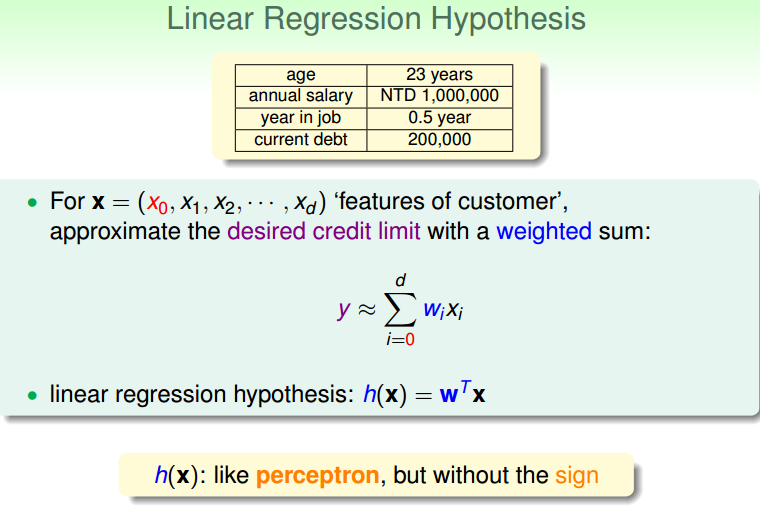h(x)=w^TX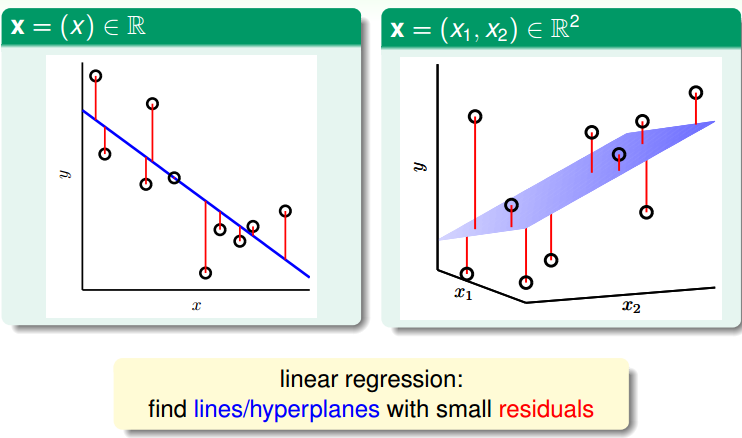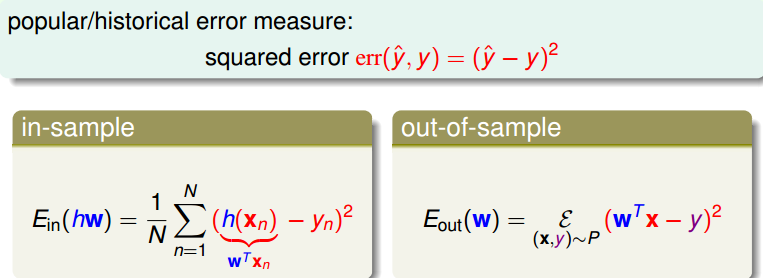## 二、线性回归算法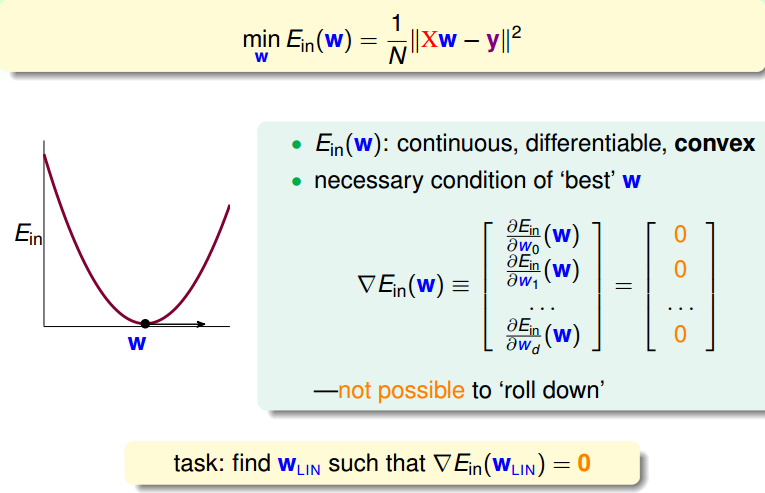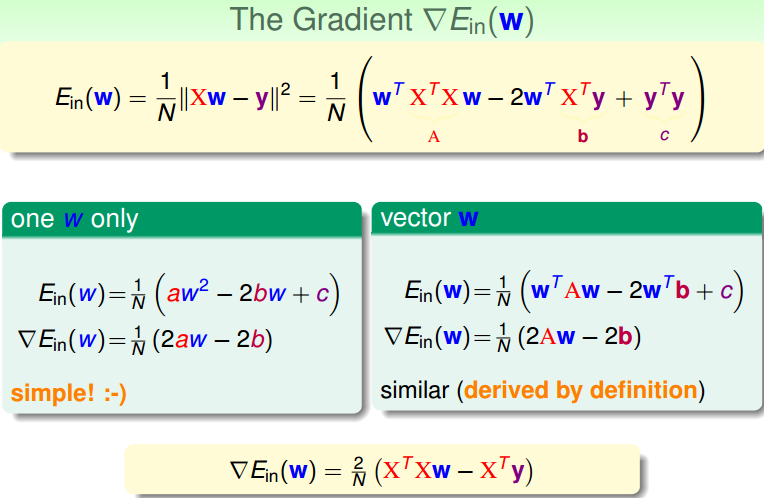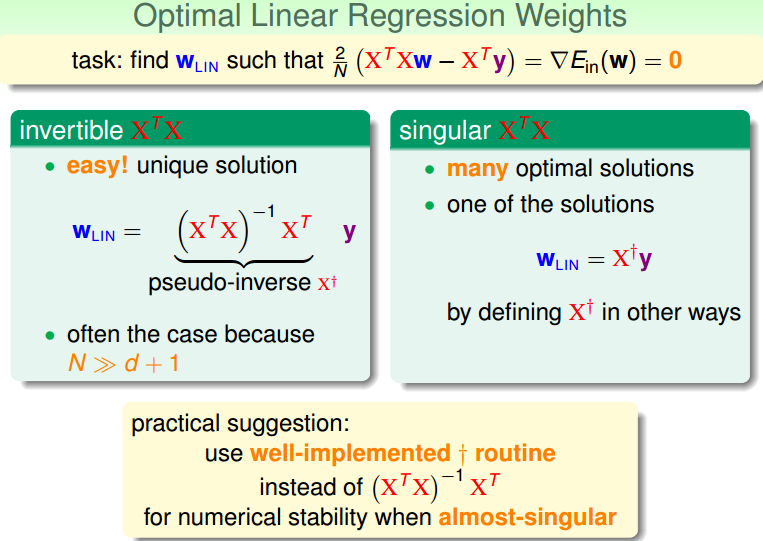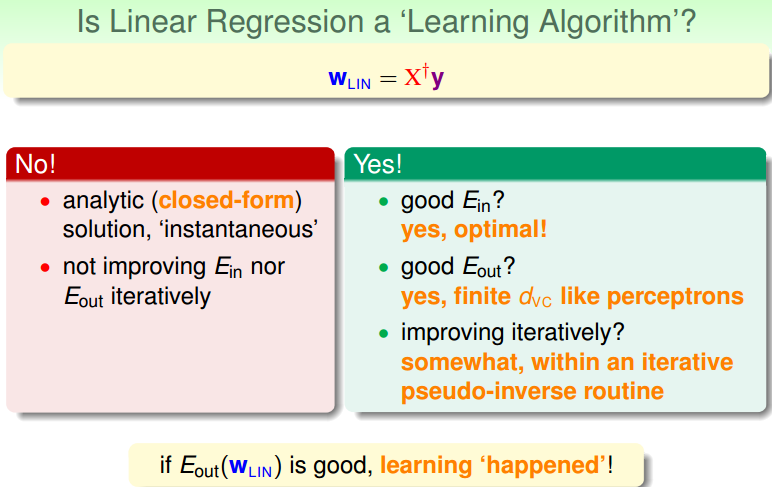## 三、泛化问题trace(I-H)=trace(I)-trace(H)
=N-trace(XX^+)=N-trace(X(X^TX)^{-1}X^T
=N-trace(X^TX(X^TX)^{-1})=N-trace(I_{d+1})
=N-(d+1)

## 四、Linear Regression方法解决Linear Classification问题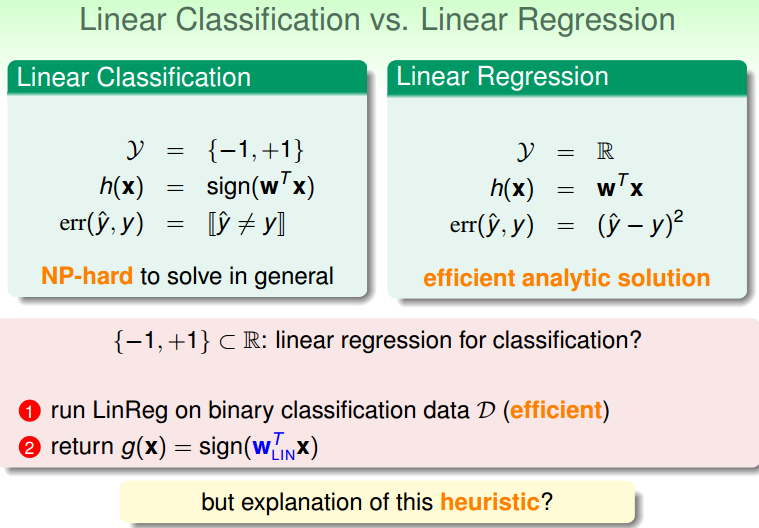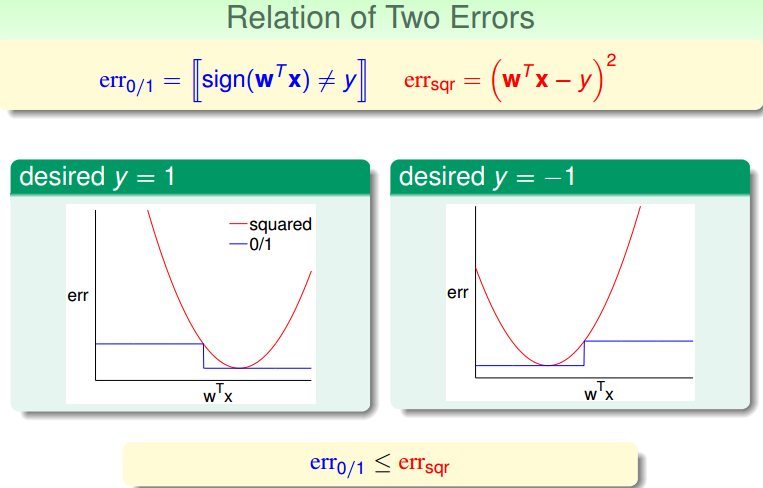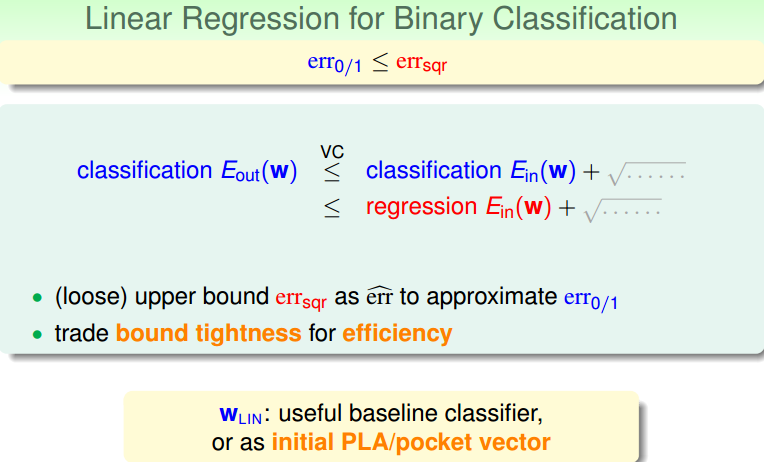## 五、总结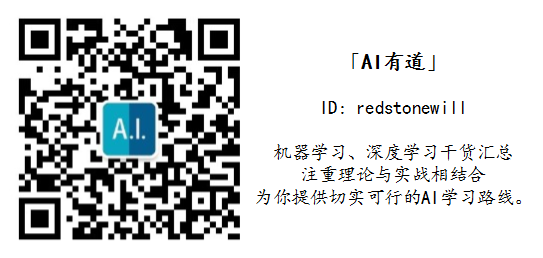### 觉得文章有用就打赏一下文章作者

#### 支付宝扫一扫打赏#### 微信扫一扫打赏